# R S Aggarwal Solutions for Class 11 Maths Chapter 28 Differentiation Exercise 28E

Exercise 28E of Chapter 28 is based on topic “Derivative of a function of a function” or “Chain Rule”, which states that, if y = f(t) and t = g(x) then dy/dx = (dy/dt x dt/dx). This rule can also be extended as: If y = f(t), t = g(u) and u = h(x) then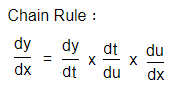Class 11 Maths Chapter 28 Differentiation Exercise 28E is based on the topic: Derivative of a function of a function. The step by step solution in R S Aggarwal Solution for Class 11 Mathematics offers invaluable help while doing homework and preparing for exams.

## Download PDF of R S Aggarwal Solutions for Class 11 Maths Chapter 28 Differentiation Exercise 28E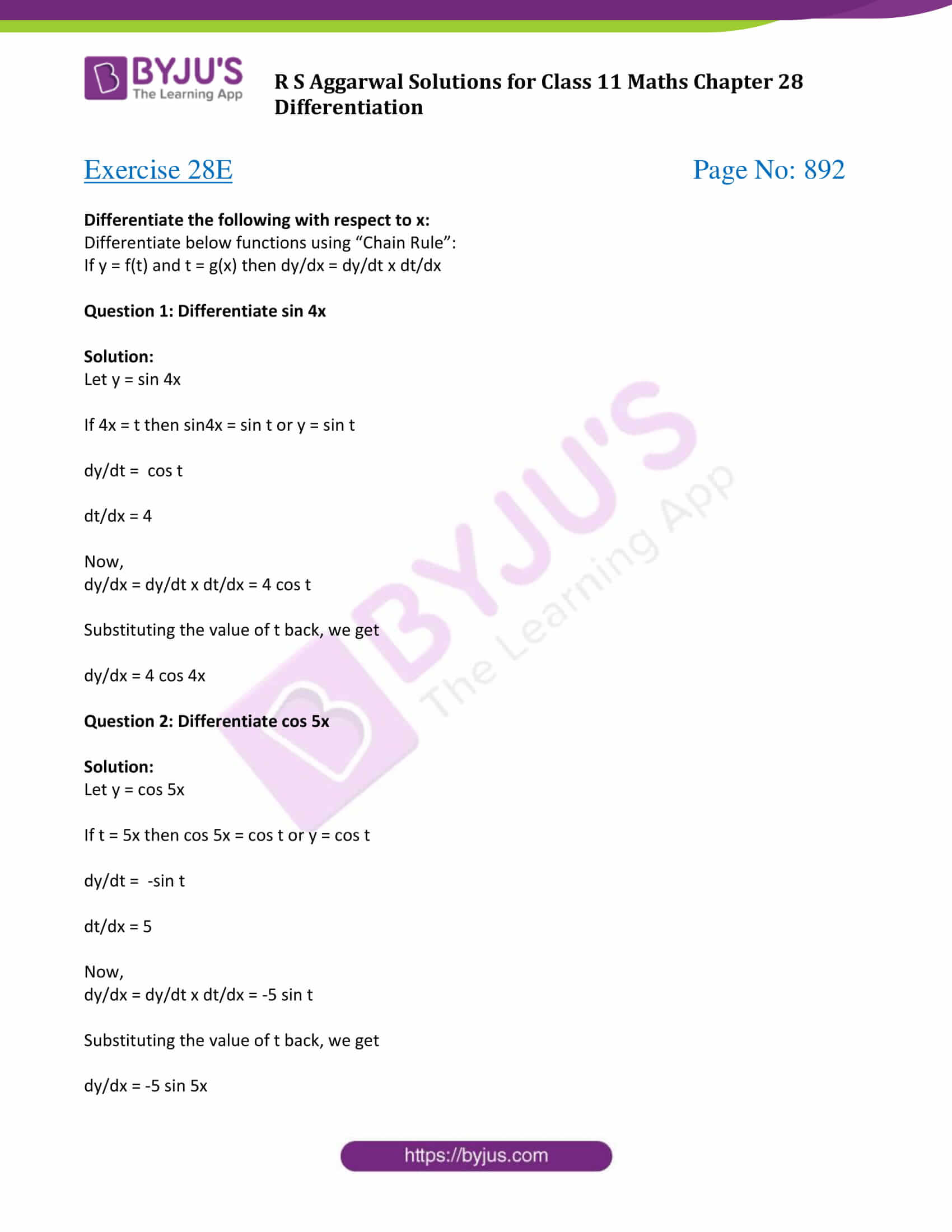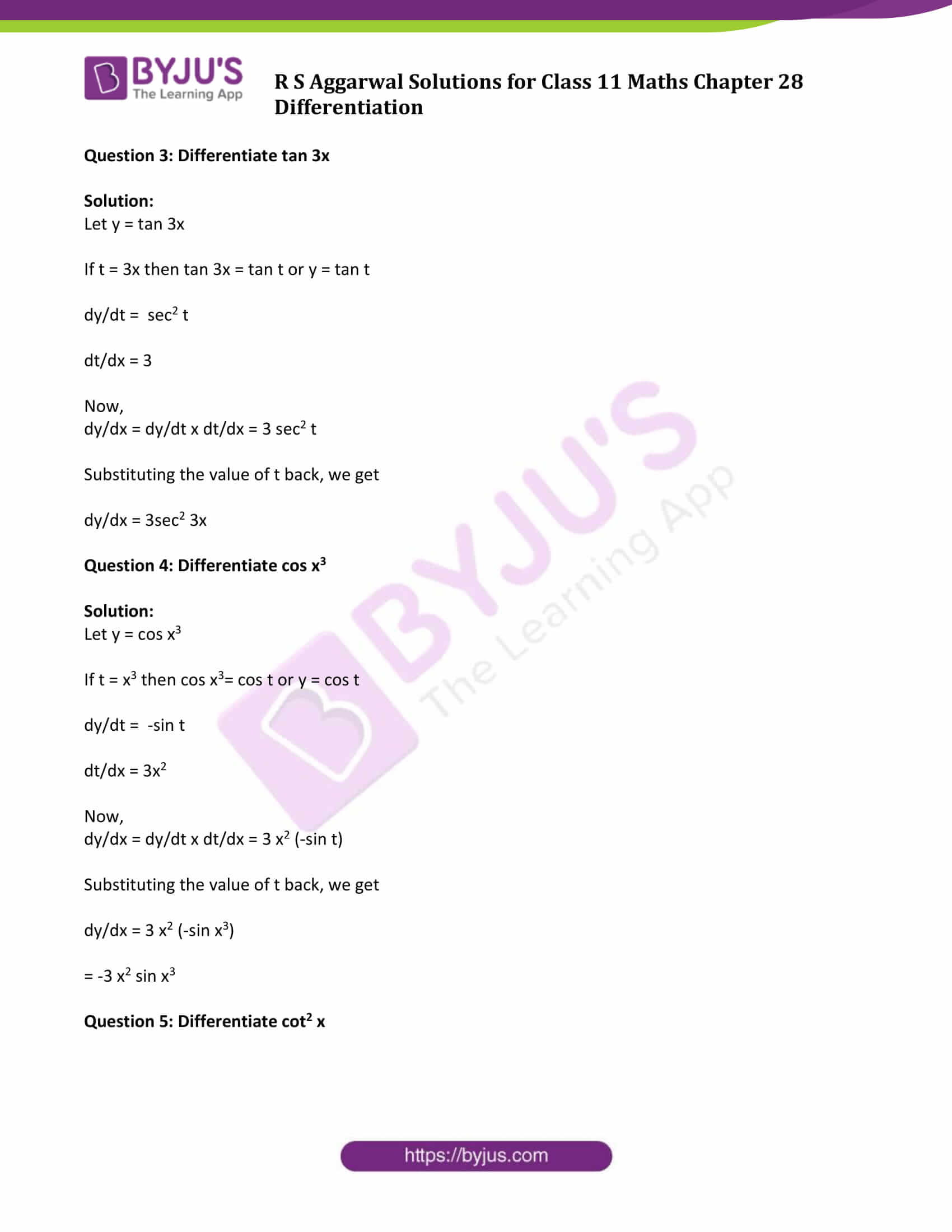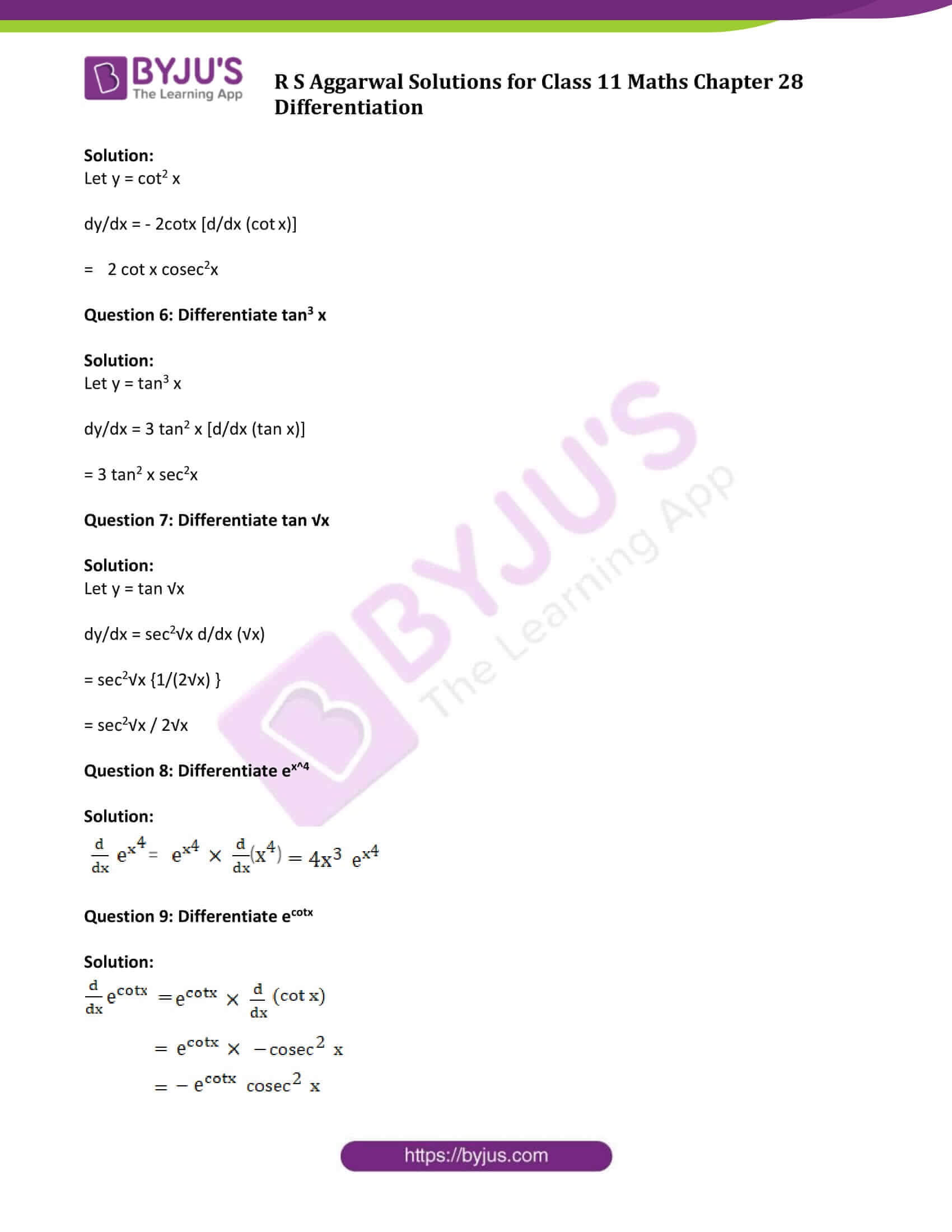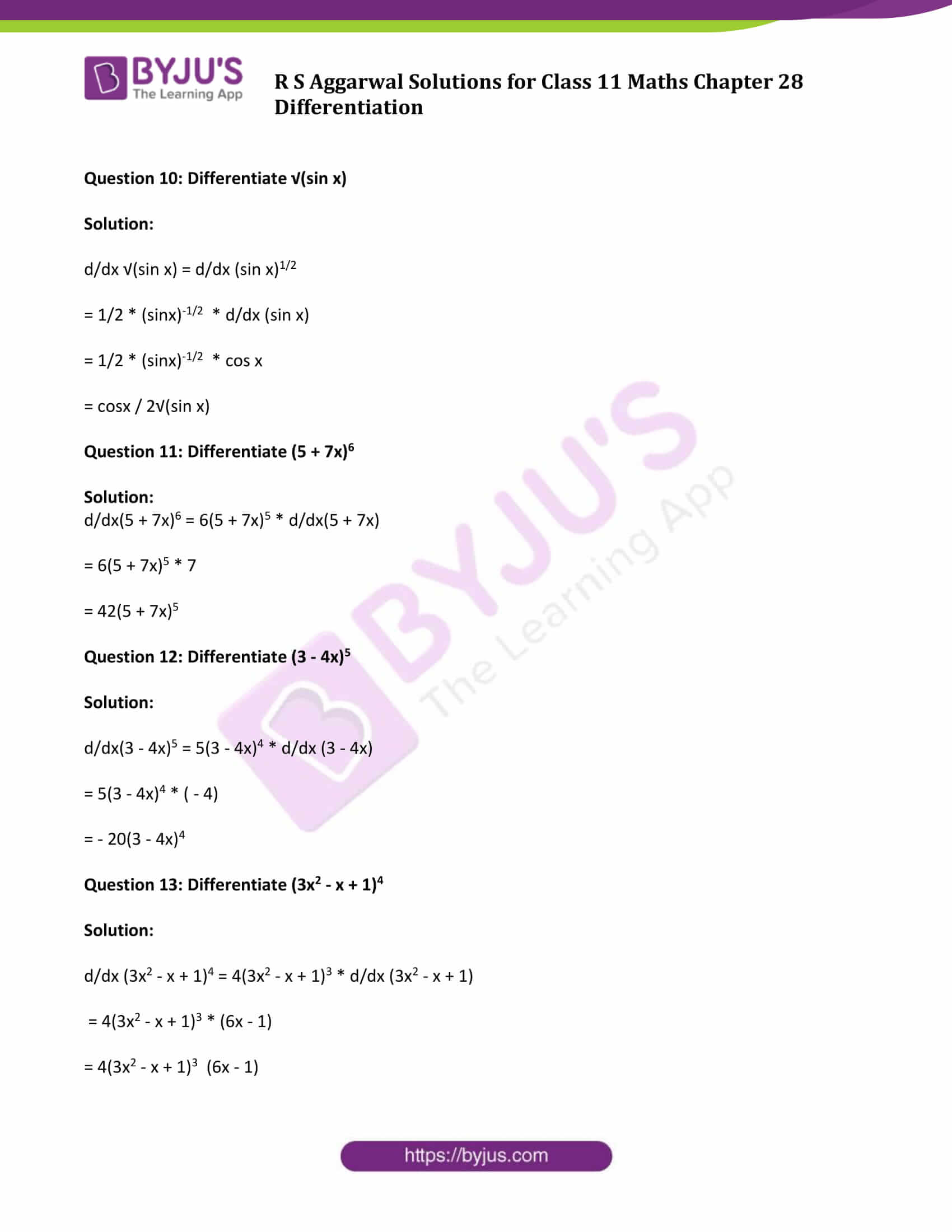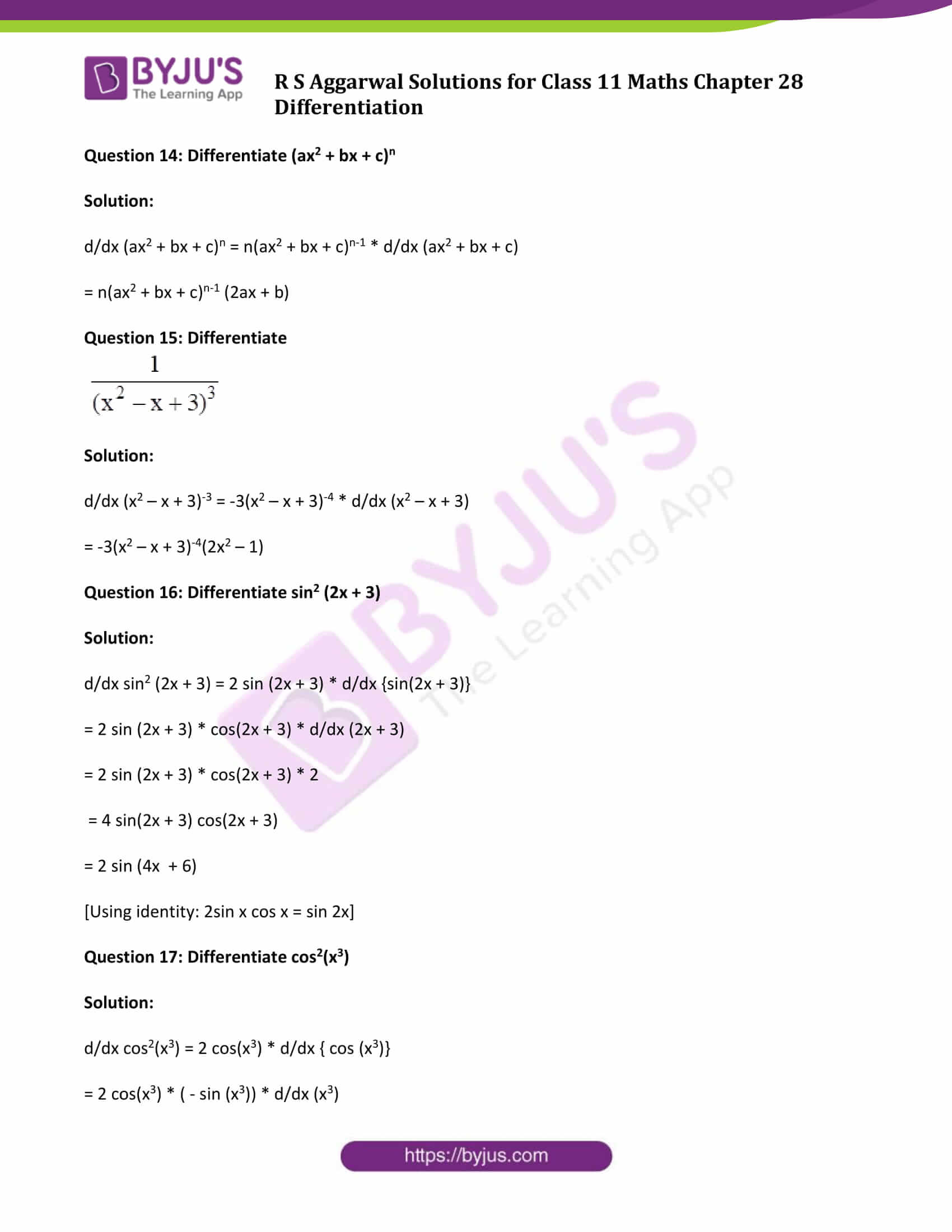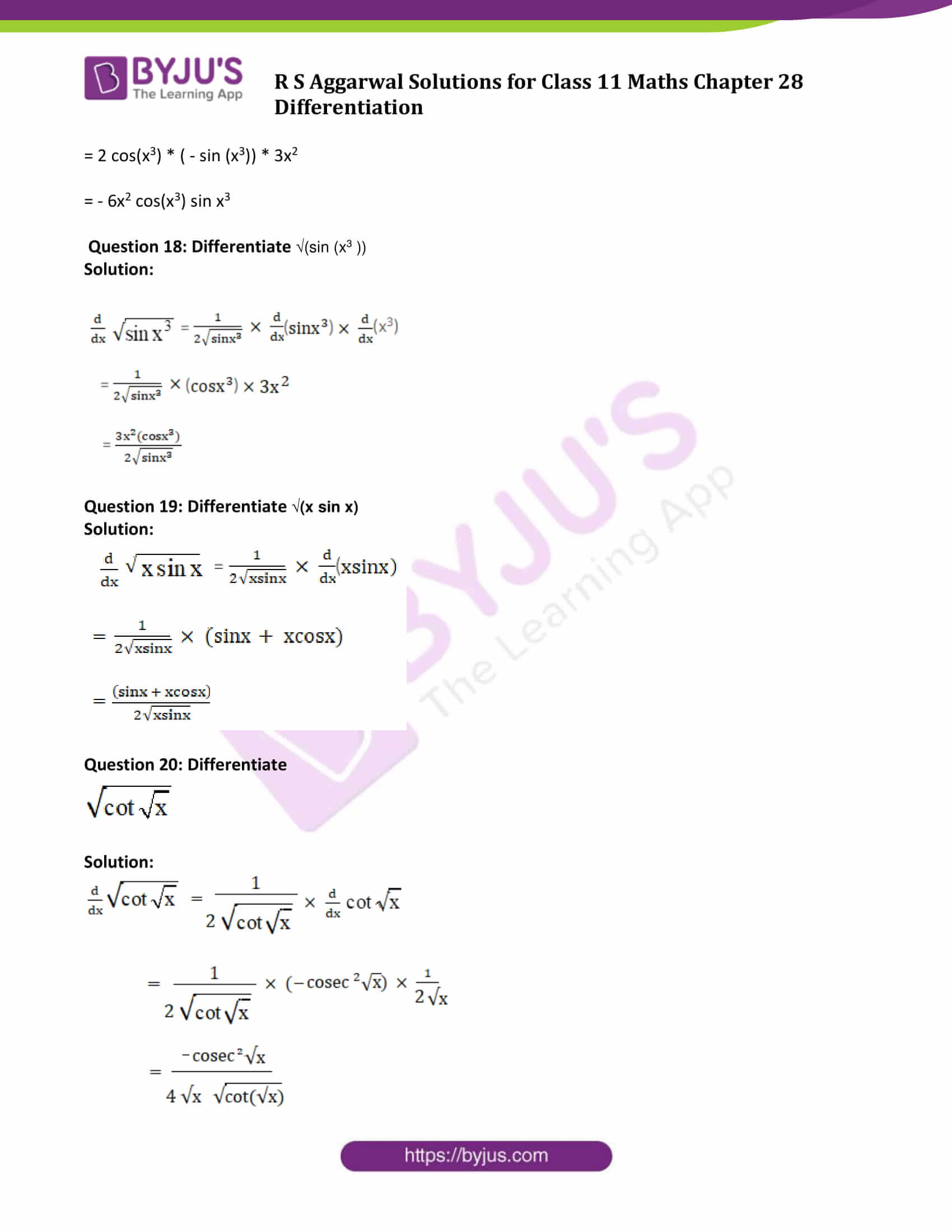### Access Answers to Maths R S Aggarwal Class 11 Chapter 28 Differentiation Exercise 28E Page number 892

Differentiate the following with respect to x:

Differentiate below functions using “Chain Rule”:

If y = f(t) and t = g(x) then dy/dx = dy/dt x dt/dx

Question 1: Differentiate sin 4x

Solution:

Let y = sin 4x

If 4x = t then sin4x = sin t or y = sin t

dy/dt = cos t

dt/dx = 4

Now,

dy/dx = dy/dt x dt/dx = 4 cos t

Substituting the value of t back, we get

dy/dx = 4 cos 4x

Question 2: Differentiate cos 5x

Solution:

Let y = cos 5x

If t = 5x then cos 5x = cos t or y = cos t

dy/dt = -sin t

dt/dx = 5

Now,

dy/dx = dy/dt x dt/dx = -5 sin t

Substituting the value of t back, we get

dy/dx = -5 sin 5x

Question 3: Differentiate tan 3x

Solution:

Let y = tan 3x

If t = 3x then tan 3x = tan t or y = tan t

dy/dt = sec2 t

dt/dx = 3

Now,

dy/dx = dy/dt x dt/dx = 3 sec2 t

Substituting the value of t back, we get

dy/dx = 3sec2 3x

Question 4: Differentiate cos x3

Solution:

Let y = cos x3

If t = x3 then cos x3= cos t or y = cos t

dy/dt = -sin t

dt/dx = 3x2

Now,

dy/dx = dy/dt x dt/dx = 3 x2 (-sin t)

Substituting the value of t back, we get

dy/dx = 3 x2 (-sin x3)

= -3 x2 sin x3

Question 5: Differentiate cot2 x

Solution:

Let y = cot2 x

dy/dx = – 2cotx [d/dx (cot x)]

= 2 cot x cosec2x

Question 6: Differentiate tan3 x

Solution:

Let y = tan3 x

dy/dx = 3 tan2 x [d/dx (tan x)]

= 3 tan2 x sec2x

Question 7: Differentiate tan √x

Solution:

Let y = tan √x

dy/dx = sec2√x d/dx (√x)

= sec2√x {1/(2√x) }

= sec2√x / 2√x

Question 8: Differentiate ex^4

Solution: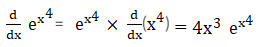Question 9: Differentiate ecotx

Solution:Question 10: Differentiate √(sin x)

Solution:

d/dx √(sin x) = d/dx (sin x)1/2

= 1/2 * (sinx)-1/2 * d/dx (sin x)

= 1/2 * (sinx)-1/2 * cos x

= cosx / 2√(sin x)

Question 11: Differentiate (5 + 7x)6

Solution:

d/dx(5 + 7x)6 = 6(5 + 7x)5 * d/dx(5 + 7x)

= 6(5 + 7x)5 * 7

= 42(5 + 7x)5

Question 12: Differentiate (3 – 4x)5

Solution:

d/dx(3 – 4x)5 = 5(3 – 4x)4 * d/dx (3 – 4x)

= 5(3 – 4x)4 * ( – 4)

= – 20(3 – 4x)4

Question 13: Differentiate (3x2 – x + 1)4

Solution:

d/dx (3x2 – x + 1)4 = 4(3x2 – x + 1)3 * d/dx (3x2 – x + 1)

= 4(3x2 – x + 1)3 * (6x – 1)

= 4(3x2 – x + 1)3 (6x – 1)

Question 14: Differentiate (ax2 + bx + c)n

Solution:

d/dx (ax2 + bx + c)n = n(ax2 + bx + c)n-1 * d/dx (ax2 + bx + c)

= n(ax2 + bx + c)n-1 (2ax + b)

Question 15: Differentiate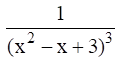Solution:

d/dx (x2 – x + 3)-3 = -3(x2 – x + 3)-4 * d/dx (x2 – x + 3)

= -3(x2 – x + 3)-4(2x2 – 1)

Question 16: Differentiate sin2 (2x + 3)

Solution:

d/dx sin2 (2x + 3) = 2 sin (2x + 3) * d/dx {sin(2x + 3)}

= 2 sin (2x + 3) * cos(2x + 3) * d/dx (2x + 3)

= 2 sin (2x + 3) * cos(2x + 3) * 2

= 4 sin(2x + 3) cos(2x + 3)

= 2 sin (4x + 6)

[Using identity: 2sin x cos x = sin 2x]

Question 17: Differentiate cos2(x3)

Solution:

d/dx cos2(x3) = 2 cos(x3) * d/dx { cos (x3)}

= 2 cos(x3) * ( – sin (x3)) * d/dx (x3)

= 2 cos(x3) * ( – sin (x3)) * 3x2

= – 6x2 cos(x3) sin x3

Question 18: Differentiate √(sin (x3 ))

Solution: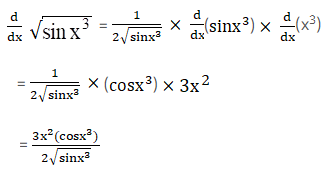Question 19: Differentiate √(x sin x)

Solution: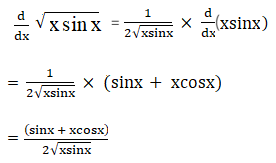Question 20: Differentiate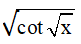Solution: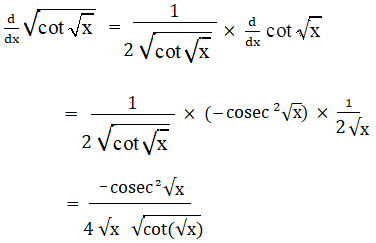## Access other exercise solutions of Class 11 Maths Chapter 28 Differentiation

Exercise 28A Solutions

Exercise 28B Solutions

Exercise 28C Solutions

Exercise 28D Solutions